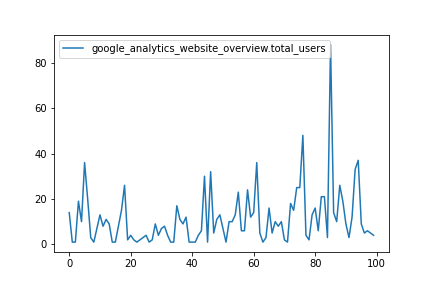# Fetch Data into a Jupyter Notebook

• Time required: 5 minutes

## Prerequisites

• Signed up for a Matatika account
• Created a workspace through the Matatika app or API

Refer to the previous Getting Started guides if you are unsure of these requirements.

## Introduction

Each dataset has a `data` endpoint, which returns live data from the database workspace schema based on the dataset `query`. The Matatika library `fetch` method is used to tap into this endpoint and return a snapshot of the dataset data. Using a Jupyter Notebook, we can create an interactive environment to fetch some data and perform transform and visualisation operations.

You can follow along with this guide using our simple_jupyter_fetch example notebook.

## Fetching Data

Dataset data can be retrieved by invoking `fetch` as follows:

``````from matatika.library import MatatikaClient

# create the client and call 'fetch'
client = MatatikaClient(auth_token, endpoint_url, None)
data = client.fetch(dataset_id)
``````

By default, the method will return a Python dictionary object constructed from the raw API response. From here, with the use of data-centric libraries such as pandas, NumPy or SciPy, it becomes easy to begin analysing, transforming and visualising the data in useful ways.

## Using the Data

We can create a `pandas.DataFrame` using the `from_dict` method, and supplying the Matatika client-library `fetch` method return value as the argument.

``````import pandas as pd

# create the dataframe from the dataset data dictionary
df = pd.DataFrame.from_dict(data)
The resulting dataframe can be visualised using the `plot` method, which functions as a wrapper for the plotting backend (by default this is Matplotlib).
``````df.plot()